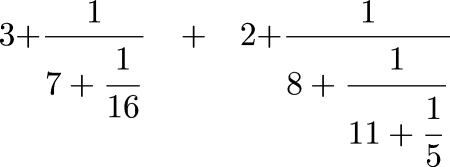Next Continued Fraction Arithmetic 23

# The catch

• How the heck do you do arithmetic on continued fractions?

• How do you add, subtract, multiply and divide them?• Or consider this:

```        0    = 
1/8  = [0; 8]
2/8  = [0; 4]
3/8  = [0; 2, 1, 2]
4/8  = [0; 2]
5/8  = [0; 1, 1, 1, 2]
6/8  = [0; 1, 3]
7/8  = [0; 1, 7]
1    = 
```
• It seems that the only way to evaluate this is to turn both addends into rational numbers

• Which defeats the whole purpose

• And anyway doesn't work for infinite continued fractions

 Next Back